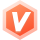weixin_51337768 2021-05-29 18:10 采纳率: 20%

# 有向图十字链表的创立，能运行但是输入边数和顶点数就突然结束运行了，求大神救救谢谢了

``````代码是在devc++运行的，我试了好久还是这样救命啊

#include <stdio.h>
#include <stdlib.h>

typedef struct Arcbox//弧
{
int weight;
}Arcbox;

typedef struct Vexbox//顶点
{
char data;
Arcbox *firstin,*firstout;
}Vexbox;

typedef struct Graph//总 //结构体名不能为空
{
Vexbox xlist;
int vexnum,arcnum;
}*LGraph;

void creatG(LGraph G)
{
int i;
printf("请输入顶点数和边数:\n");
scanf("%d %d",&G->vexnum,&G->arcnum);
for(i=0;i<G->vexnum;i++)
{
G->xlist[i].firstin=NULL;
G->xlist[i].firstout=NULL;
}
printf("请连续输入顶点名称：\n");
for(i=0;i<G->vexnum;i++)
{
scanf("%c",&G->xlist[i].data);
}
/*for(i=0;i<G->vexnum;i++)
{
printf("请输入第%d个顶点的值：",i+1);
scanf("%c",&G->xlist[i].data);
G->xlist[i].firstin=G->xlist[i].firstout=NULL;
}*/
int j,k;
char b,c;
int w;
for(i=0;i<G->arcnum;i++)
{
printf("请输入边的两个顶点和权值\n");
scanf("%c %c %d",&b,&c,&w);
for(j=0;j<G->vexnum;j++)
{
if(G->xlist[j].data==b)
{
break;
}
}
for(k=0;k<G->vexnum;k++)
{
if(G->xlist[k].data==c)
{
break;
}
}
//出弧操作
Arcbox *p1,*p2;
p1=(Arcbox*)malloc(sizeof(struct Arcbox)) ;
p1->tailvex=k;
p1->weight=w;
G->xlist[j].firstout=p1;
//入弧操作
if(G->xlist[k].firstin==NULL)
{
G->xlist[k].firstin=p1;
}
else
{
p2=G->xlist[k].firstin;
{
}
}
}
}

void inputG(LGraph G)
{
printf("十字链表为：\n");
int i;
Arcbox *p;
//出弧
for(i=0;i<G->vexnum;i++)
{
printf("%c",G->xlist[i].data);
p=G->xlist[i].firstout;
while(p)
{
}
printf("\n");
}
//入弧
for(i=0;i<G->vexnum;i++)
{
printf("%c",G->xlist[i].data);
p=G->xlist[i].firstin;
while(p)
{
}
printf("\n");
}
}

int main()
{
LGraph 	G;
creatG(G);
inputG(G);
return 0;
} ``````
• 写回答

#### 1条回答默认 最新

•泡沫o0 C/C++ 领域优质创作者 2023-06-11 14:10
关注

你的程序突然终止可能是因为内存分配的问题。你的函数`creatG`在初始化顶点数组`xlist`时并没有为其分配内存，因此可能会导致访问非法内存并引发段错误。

`LGraph G;`只声明了一个指向Graph类型的指针，并没有为其分配内存。在C语言中，指针需要指向一块有效的内存才能被使用，因此在使用这个指针之前，你需要为它分配内存。

你可以在`main`函数中通过`malloc`函数为它分配内存，如下所示：

``````int main()
{
LGraph G = (LGraph)malloc(sizeof(struct Graph)); // 为G分配内存
creatG(G);
inputG(G);
free(G); // 使用完成后，记得释放分配的内存
return 0;
}
``````

注意，在使用`malloc`函数分配的内存使用完成后，需要用`free`函数释放，否则可能会导致内存泄露。

另一个可能的问题是输入流的处理。当你使用`scanf`读取输入时，它会在输入流中留下换行符。然后当你再次调用`scanf`读取字符时，它可能会立即读取这个换行符，导致程序的行为与你的期望不符。你可以在`scanf`之后添加一个`getchar`来消耗这个换行符，例如：

``````printf("请输入顶点数和边数:\n");
scanf("%d %d",&G->vexnum,&G->arcnum);
getchar(); // 消耗换行符
``````

应用这两个修复后，你的程序应该可以正常运行了。

评论

#### 悬赏问题

• ¥20 关于#javascript#的问题：但是我写的只能接码数字和字符，帮我写一个解码JS问题
• ¥15 prophet运行报错，如何解决？
• ¥20 前端数据是从session等作用域拿到的，如何取值继续传递后端呢
• ¥15 eclipse无法正常运行
• ¥15 定义了函数，但是无法根据函数定义触发器
• ¥20 5变量卡诺图化简得出与非门电路图
• ¥15 Python爬取交通拥堵指数数据
• ¥15 使用vba抓取重定向网页问题
• ¥20 付费需求测试程序（细谈）。
• ¥15 为什么这段c++代码会报这么多语法错误？